# 标准差分进化算法（DE）

1.初始化种群，评价出适应度值，并找出全局最优位置与最优适应度值。
2.进入优化，generation+1。
3.变异。为了保证多样性，变异中需要选择的随机粒子不应与当前更新粒子相同。变异策略通常有以下5种：

(1)DE/rand/1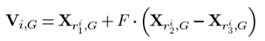(2)DE/best/1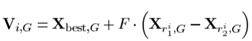Xbest是种群最优位置，在上式中有着指导作用，能够提升粒子的收敛性。

(3)DE/rand-to-best/1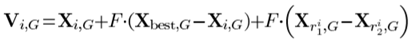(4)DE/best/2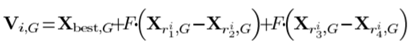(5)DE/rand/2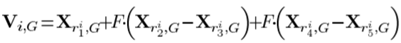4.交叉。U为交叉向量，CR是交叉概率。CR的值越大，发生交叉的概率越大，通常CR=0.3。
（1）二项式交叉

      %NP：种群个数；Dim:维度；V：变异向量；U：交叉向量；
for i=1:NP
jRand=randi([1,Dim]);  %jRand∈[1,Dim]
for j=1:Dim
k=rand;
if k<=CR||j==jRand
%j==jRand是为了确保至少有一个U(i,j)=V(i,j)
U(i,j)=V(i,j);
else
U(i,j)=X(i,j);
end
end
end


（2）指数交叉

       for i=1:NP
j=randi([1,Dim]);%j∈[1,Dim]
L=0;
U(i,:)=X(i,:);
k=rand;
while(k<CR && L<Dim)
U(i,j)=V(i,j);
j=j+1;
if(j>Dim)
j=1;
end
L=L+1;
end
end


5.评价进化的新粒子U，适应度值为fitnessU,根据U的适应度值的高低载进行以下筛选。

        if fitnessU(i)<fitnessX(i)
X(i,:)=U(i,:);
fitnessX(i)=fitnessU(i);
end


6.更新最佳位置及适应度值，进入下一代循环stesp2。

DE算法的实现比较简单，算法的灵活性较强，不同方法的变异交叉会有不同的实验效果。对于DE算法的改进也非常多，很多改进方法使得其性能突飞猛进，个人认为是一个比较普遍且适应性强的一个基础算法。

©️2019 CSDN 皮肤主题: 大白 设计师: CSDN官方博客